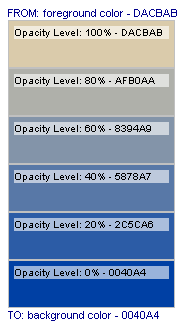GIDNetwork > Blended Colours by Opacity, a PHP Function Register

# Blended Colours by Opacity, a PHP Function

by: admin - Mar 26, 2007

A couple of days ago, I wrote this PHP function to help me find a colour by manipulating opacity levels between 2 colours. I just give the function a foreground colour, a background colour, and a suitable opacity level (for the foreground colour) and have it return a hexadecimal number.

In plain English: if the foreground colour is FFFFFF (white) and the background color is 000000 (black), and the opacity level is set at 50, I would expect the function to return 808080 (grey or gray).

If you have had any experience using popular graphics software like Adobe Photoshop, you'll recognise this feature when you create an image with 2 or more layers (filled with colour) and slide the opacity tool in the Layers window. The more you slide it to the left, the more the current layer becomes transparent (less opacity), blending the foreground with the background colour, whatever it may be.

For those who don't know, opacity means the quality of being opaque to a degree; the degree to which something reduces the passage of light. The oppposite of opacity is transparency. So, the more opaque a colour, the less transparent it is.

## PHP function to find a (blended) color by opacity

### Function: color_blend_by_opacity()

This function works only with hexadecimal colour (or color) values. It accepts the foreground and background colours in hexadecimal numbers and the opacity level in integer.

PHP Code Example:

``````
<?php

/**
* Find the resulting colour by blending 2 colours
* and setting an opacity level for the foreground colour.
*
* @author J de Silva
* @param string \$foreground Hexadecimal colour value of the foreground colour.
* @param integer \$opacity Opacity percentage (of foreground colour). A number between 0 and 100.
* @param string \$background Optional. Hexadecimal colour value of the background colour. Default is: <code>FFFFFF</code> aka white.
* @return string Hexadecimal colour value. <code>false</code> on errors.
*/
function color_blend_by_opacity( \$foreground, \$opacity, \$background=null )
{
static \$colors_rgb=array(); // stores colour values already passed through the hexdec() functions below.

if( is_null(\$background) )
\$background = 'FFFFFF'; // default background.

\$pattern = '~^[a-f0-9]{6,6}\$~i'; // accept only valid hexadecimal colour values.
if( !@preg_match(\$pattern, \$foreground)  or  !@preg_match(\$pattern, \$background) )
{
trigger_error( "Invalid hexadecimal colour value(s) found", E_USER_WARNING );
return false;
}

\$opacity = intval( \$opacity ); // validate opacity data/number.
if( \$opacity>100  || \$opacity<0 )
{
trigger_error( "Opacity percentage error, valid numbers are between 0 - 100", E_USER_WARNING );
return false;
}

if( \$opacity==100 )    // \$transparency == 0
return strtoupper( \$foreground );
if( \$opacity==0 )    // \$transparency == 100
return strtoupper( \$background );
// calculate \$transparency value.
\$transparency = 100-\$opacity;

if( !isset(\$colors_rgb[\$foreground]) )
{ // do this only ONCE per script, for each unique colour.
\$f = array(  'r'=>hexdec(\$foreground.\$foreground),
'g'=>hexdec(\$foreground.\$foreground),
'b'=>hexdec(\$foreground.\$foreground)    );
\$colors_rgb[\$foreground] = \$f;
}
else
{ // if this function is used 100 times in a script, this block is run 99 times.  Efficient.
\$f = \$colors_rgb[\$foreground];
}

if( !isset(\$colors_rgb[\$background]) )
{ // do this only ONCE per script, for each unique colour.
\$b = array(  'r'=>hexdec(\$background.\$background),
'g'=>hexdec(\$background.\$background),
'b'=>hexdec(\$background.\$background)    );
\$colors_rgb[\$background] = \$b;
}
else
{ // if this FUNCTION is used 100 times in a SCRIPT, this block will run 99 times.  Efficient.
\$b = \$colors_rgb[\$background];
}

\$add = array(    'r'=>( \$b['r']-\$f['r'] ) / 100,
'g'=>( \$b['g']-\$f['g'] ) / 100,
'b'=>( \$b['b']-\$f['b'] ) / 100    );

\$f['r'] += intval( \$add['r'] * \$transparency );
\$f['g'] += intval( \$add['g'] * \$transparency );
\$f['b'] += intval( \$add['b'] * \$transparency );

return sprintf( '%02X%02X%02X', \$f['r'], \$f['g'], \$f['b'] );
}
\?>

``````

Although it is not immediately obvious, a function like this will be used multiple times within a script. If you are going to have any real use for this function, it is quite likely that you will be calling it up to 2 times or more in the script, e.g. within a loop. You'll see what I mean, when you see the example script below.

For this reason alone, I put some extra effort into the function to make it work more efficiently. The function saves each colour that has been passed through the hexdec() function into a static array so it does not process the duplicate colour on each instance, within a script.

Here is a simple script you can copy, paste, and run. It demonstrates how you can use this function. It will also help you decide if this is something you can use in your own web applications.

PHP Code Example:

``````
<?php
//* Fill-in 2 random colour values below.
\$foreground = 'DACBAB';
\$background = '0040A4'; //*/
?><html>
<title>Test PHP Function: color_blend_by_opacity()</title>
<style type="text/css"><!--
div.outer { border:1px solid silver; font:normal 10px arial,sans-serif; height:36px; padding:5px; width:155px; }
div.titles { color:darkblue; font:normal 11px arial,sans-serif; }
--></style>
<body><?php

//*
echo "<div class='titles'>FROM: foreground color - \$foreground</div>";
for( \$i=100; \$i>=0; \$i-=20 )
{
if( \$o_color=@color_blend_by_opacity(\$foreground, \$i, \$background) )
{
printf( '<div class="outer" style="background-color:#%1\$s;">'.
'<div style="background-color:#%3\$s;">Opacity Level: %2\$d%% - %1\$s</div></div>',
\$o_color, \$i, @color_blend_by_opacity('FFFFFF', 60, \$o_color) );
}
else
{
echo '<p>Error</p>';
}
}
echo "<div class='titles'>TO: background color - \$background</div>"; //*/
?>
</body>
</html>
<?php

// Function

/**
* Find the resulting colour by blending 2 colours
* and setting an opacity level for the foreground colour.
*
* @author J de Silva
* @param string \$foreground Hexadecimal colour value of the foreground colour.
* @param integer \$opacity Opacity percentage (of foreground colour). A number between 0 and 100.
* @param string \$background Optional. Hexadecimal colour value of the background colour. Default is: <code>FFFFFF</code> aka white.
* @return string Hexadecimal colour value. <code>false</code> on errors.
*/
function color_blend_by_opacity( \$foreground, \$opacity, \$background=null )
{
static \$colors_rgb=array(); // stores colour values already passed through the hexdec() functions below.

if( is_null(\$background) )
\$background = 'FFFFFF'; // default background.

\$pattern = '~^[a-f0-9]{6,6}\$~i'; // accept only valid hexadecimal colour values.
if( !@preg_match(\$pattern, \$foreground)  or  !@preg_match(\$pattern, \$background) )
{
trigger_error( "Invalid hexadecimal colour value(s) found", E_USER_WARNING );
return false;
}

\$opacity = intval( \$opacity ); // validate opacity data/number.
if( \$opacity>100  || \$opacity<0 )
{
trigger_error( "Opacity percentage error, valid numbers are between 0 - 100", E_USER_WARNING );
return false;
}

if( \$opacity==100 )    // \$transparency == 0
return strtoupper( \$foreground );
if( \$opacity==0 )    // \$transparency == 100
return strtoupper( \$background );
// calculate \$transparency value.
\$transparency = 100-\$opacity;

if( !isset(\$colors_rgb[\$foreground]) )
{ // do this only ONCE per script, for each unique colour.
\$f = array(  'r'=>hexdec(\$foreground.\$foreground),
'g'=>hexdec(\$foreground.\$foreground),
'b'=>hexdec(\$foreground.\$foreground)    );
\$colors_rgb[\$foreground] = \$f;
}
else
{ // if this function is used 100 times in a script, this block is run 99 times.  Efficient.
\$f = \$colors_rgb[\$foreground];
}

if( !isset(\$colors_rgb[\$background]) )
{ // do this only ONCE per script, for each unique colour.
\$b = array(  'r'=>hexdec(\$background.\$background),
'g'=>hexdec(\$background.\$background),
'b'=>hexdec(\$background.\$background)    );
\$colors_rgb[\$background] = \$b;
}
else
{ // if this FUNCTION is used 100 times in a SCRIPT, this block will run 99 times.  Efficient.
\$b = \$colors_rgb[\$background];
}

\$add = array(    'r'=>( \$b['r']-\$f['r'] ) / 100,
'g'=>( \$b['g']-\$f['g'] ) / 100,
'b'=>( \$b['b']-\$f['b'] ) / 100    );

\$f['r'] += intval( \$add['r'] * \$transparency );
\$f['g'] += intval( \$add['g'] * \$transparency );
\$f['b'] += intval( \$add['b'] * \$transparency );

return sprintf( '%02X%02X%02X', \$f['r'], \$f['g'], \$f['b'] );
}
?>

``````

If you didn't edit the \$foreground and \$background values in the script, the resulting web page would display something like this:I can imagine quite a few uses for this function. You can use it to display "disabled" or "inactive" rows of data, for example, i.e by 'fading' (50% opacity) the regular background and font colors of the normal rows. Display web site menus by giving it a 'gradient' look. You could also lighten a colour by setting the background color to white and setting a suitable opacity level. These are just a few ideas I can think of right now, as I write this. :)

As usual, I will appreciate your feedback, if you have any.

Also, thank you, Mark, for pushing me in the right direction! :)

 Would you like to comment? This story has been viewed 37,224 times.

__top__